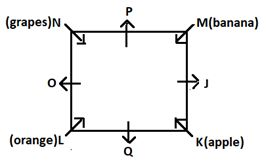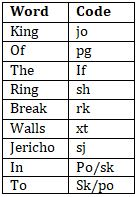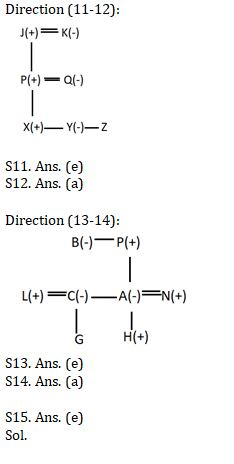Latest Banking jobs   »   Reasoning Ability Quiz For IBPS Clerk...

# Reasoning Ability Quiz For IBPS Clerk Prelims 2021- 24th August

Directions (1-5): Study the following information carefully and answer the questions given below:
Eight persons i.e. J, K, L, M, N, O, P and Q sits around a Square table with four of them sitting around the corner and are facing towards the centre of table while rest are sitting around the middle side of table and are facing away from the centre of table. There is equal distance between all persons. Those sitting at the corner of table like a different fruit i.e. Apple, Orange, Grape and Banana.
N sits third to the left of J. K sits to the immediate left of Q. M sits opposite to L, who does not sit at middle of the sides. J is immediate neighbor of K. L does not sit next to P. Person sitting next to O likes either Grape or Orange. Three persons sit between Q and P. The one who likes Banana sits second to the left of the one who likes Grape.

Q1. Who among the following faces the one who likes Banana?
(a) O
(b) N
(c) The one who likes Orange
(d) The one who likes Grape
(e) None of these

Q2. Who among the following faces J?
(a) O
(b) M
(c) The one who likes Apple
(d) L
(e) None of these

Q3. The number of person sitting between the one who likes Grape and M, when counted from the right of M is same as the number of persons sitting between the one who likes Apple and ____, when counted from the right of ____?
(a) M
(b) J
(c) The one who likes Orange
(d) Q
(e) None of these

Q4. Who among the following likes Banana?
(a) J
(b) L
(c) M
(d) The one who faces N
(e)None of these

Q5. Four of the following are alike in a certain way and hence they form a group. Which one of the following does not belong to that group?
(a) N
(b) K
(c) M
(d) L
(e) O

Direction (6-10): Study the following information carefully and answer the questions given below:
In a certain code:
“King of the Ring” is coded as “jo pg if sh”
“Ring in to Jericho” is coded as “sh po sk sj”
“Walls of Jericho” is coded as “xt pg sj”
“Break the Walls” is coded as “rk if xt”

Q6. What is the code for the word “in”?
(a) jo
(b) pg
(c) po
(d) sk
(e) Cannot be determined

Q7. What is the code for the word “Break”?
(a) rk
(b) jo
(c) po
(d) sk
(e) None of the above

Q8. What is the code for the word “to”?
(a) jo
(b) pg
(c) po
(d) sk
(e) Cannot be determined

Q9. What is the code for the word “Jericho”?
(a) sk
(b) sh
(c) sj
(d) rk
(e) None of the above

Q10. Which of the following word is coded as “xt”?
(a) in
(b) Break
(c) Jericho
(d) Walls
(e) None of the above

Directions (11-12): Study the following information carefully and answer the questions given below:
In a family of three generations there are seven family members i.e. J, K, P, Q, X, Y and Z. J has only one child. X is the brother of Y and Z. Y is the granddaughter of K. K is the mother-in-law of Q. P is the father of Z and son of J.

Q11. How is Z related to J?
(a) Grandson
(b) Granddaughter
(c) Father
(d) Son
(e) Cannot be determined

Q12. How is Q related to X?
(a) Mother
(b) Father
(c) Daughter
(d) Son
(e) None of these

Directions (13-14): Study the following information carefully and answer the questions given below:
In a family of three generations there are eight members i.e. P, B, N, A, C, L, H and G. B is the wife of P. H is the grandson of B. P has only two daughters. G is the cousin of H. N is the father of H. A is daughter of P. L is the son in law of B. N is husband of A. C is the aunt of H.

Q13. How is G related to B?
(a) Son
(b) Daughter
(c) Grand daughter
(d) Grand son
(e) Cannot be determined

Q14. How is L related to G?
(a) Father
(b) Mother
(c) Son
(d) Grand father
(e) Cannot be determined

Q15. How many pairs of letters are there in the word ‘TROLLS’, each of which have as many letters between them in the word as they have between them in the English alphabet?
(a) one
(b) two
(c) three
(d) four
(e) None

Solutions:

Solutions (1-5):S1. Ans. (c)
S2. Ans. (a)
S3. Ans. (c)
S4. Ans. (c)
S5. Ans. (e)

Solutions (6-10):S6. Ans. (e)
S7. Ans. (a)
S8. Ans. (e)
S9. Ans. (c)
S10. Ans. (d)